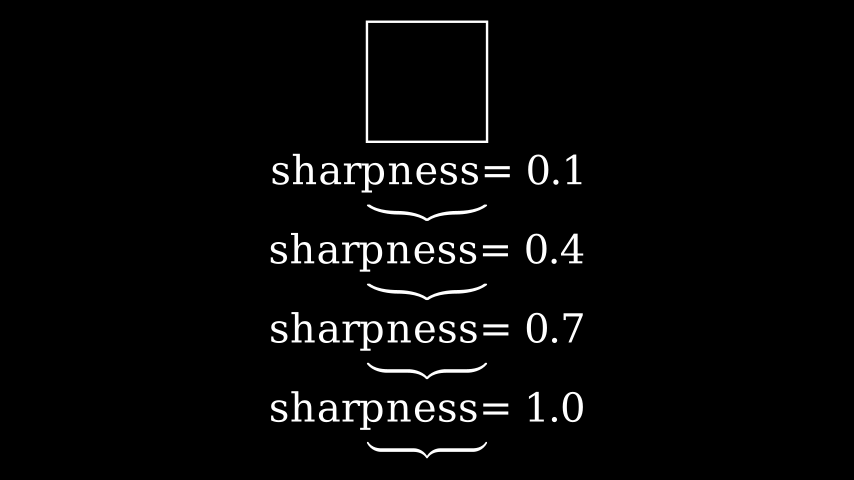# Brace#

Qualified name: `manim.mobject.svg.brace.Brace`

class Brace(mobject, direction=array([0., - 1., 0.]), buff=0.2, sharpness=2, stroke_width=0, fill_opacity=1.0, background_stroke_width=0, background_stroke_color='#000000', **kwargs)[source]#

Takes a mobject and draws a brace adjacent to it.

Passing a direction vector determines the direction from which the brace is drawn. By default it is drawn from below.

Parameters
• mobject (Mobject) – The mobject adjacent to which the brace is placed.

• direction (Sequence[float] | None) – The direction from which the brace faces the mobject.

Examples

Example: BraceExample```from manim import *

class BraceExample(Scene):
def construct(self):
s = Square()
for i in np.linspace(0.1,1.0,4):
br = Brace(s, sharpness=i)
t = Text(f"sharpness= {i}").next_to(br, RIGHT)
VGroup(*self.mobjects).arrange(DOWN, buff=0.2)
```

Methods

 `get_direction` Uses `shoelace_direction()` to calculate the direction. `get_tex` `get_text` `get_tip` `put_at_tip`

Attributes

 `animate` Used to animate the application of any method of `self`. `animation_overrides` `color` `depth` The depth of the mobject. `fill_color` If there are multiple colors (for gradient) this returns the first one `height` The height of the mobject. `n_points_per_curve` `sheen_factor` `stroke_color` `width` The width of the mobject.
get_direction()[source]#

Uses `shoelace_direction()` to calculate the direction. The direction of points determines in which direction the object is drawn, clockwise or counterclockwise.

Examples

The default direction of a `Circle` is counterclockwise:

```>>> from manim import Circle
>>> Circle().get_direction()
'CCW'
```
Returns

Either `"CW"` or `"CCW"`.

Return type

`str`